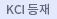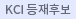#검색
다국어 입력

http://chineseinput.net/에서 pinyin(병음)방식으로 중국어를 변환할 수 있습니다.

변환된 중국어를 복사하여 사용하시면 됩니다.

예시)
• 中文 을 입력하시려면 zhongwen을 입력하시고 space를누르시면됩니다.
• 北京 을 입력하시려면 beijing을 입력하시고 space를 누르시면 됩니다.
닫기

검색결과 좁혀 보기

• 좁혀본 항목 보기순서

• 원문유무
• 음성지원유무
• 원문제공처
• 등재정보
• 학술지명
• 주제분류
• 발행연도
• 작성언어
• 저자

오늘 본 자료

• 오늘 본 자료가 없습니다.
더보기
• 무료
• 기관 내 무료
• 유료
•제천규석으로부터 제조한 $\beta$-SiC분말 및 소결체의 특성

${\beta}$-SiC powders were prepared by the simultaneous reduction and carbiding of Jecheon quartzite at 1400$^{\circ}C$ for 7 hours in hydrogen atmosphere, using graphite or carbon black as the reducing and carbiding reagent. The prepared SiC powder was acid-treated with the mixture of fluoric acid and hydrochloric acid at room temperature and also by heating on an alcohol lamp for one hour, respectively. The impurities were mostly eliminated and the purity of SiC became 98.5% after hot acid treatment. The specific surface area of SiC powder was also increased up to 115㎡/g by hot acid treatment. This pure and fine SiC powder was hot-pressed at 1900$^{\circ}C$ for 30min, using 5wt% Al2O3 as a sintering aid. The density, M.O.R., KIC and hardness of the hot-pressed SiC ceramics were 3.195g/㎤, 48.7Kgf/$\textrm{mm}^2$, 5.4MN/㎥/2 and 2,182Kgf/$\textrm{mm}^2$, respectively.

•완도납석으로부터 제조된$\beta$-Sialon의 기계적 성질

$\beta$-Sialon powders were prepared by the reduction-nitridation reaction from the mixture of Wando-pyrophyllite and graphite at 135$0^{\circ}C$ in $N_2-H_2$ atmosphere. The $\beta$-Sialon powders were sintered at 175$0^{\circ}C$ by the pressureless sintering for 90min and the hot-pressing for 60 min respectively. All the sintered bodies showed their relative densities higher than 94% The values of M.O.R fracture toughness(KIC) and hardness showed 32.9kpsi 2.9MN/$m^{1.5}$ , 12.1GN/$m^2$ for the pressure-less sitnered bodies and 48.6kpsi, 4.6 MN/4m^{1.5}$15.3GN/$m^2$for the hot-pressed bodies respectively. •Evaluation of damage on silicon wafers using the angle lapping method and a biaxial fracture strength test The damage of sawn, lapped, etched and polished wafers was evaluated by the angle lapping method and a biaxial fracture strength test. The Si wafers for analysis were fabricated by the commercial wafering process. As the wafering process proceeded, the depth of damage (DOD) measured by the angle lapping method decreased but the fracture strength measured by the ring-on-ring test increased. As a result, a correlation between DOD and fracture strength was verified using an equation for the fracture toughness, KIC. The fracture strength obtained from the ring-on-ring test was found to be one of the representative values for the mechanical properties of a wafer as it passed through the wafering process. •완도 납석으로부터$\beta$-Sialon의 합성 (2)$eta$-Sialon powders were prepared by reduction-nitridation from mixture of Wando pyrophyllite and graphite as a reducing agent at 135$0^{\circ}C$in 80%$N_2$-20%$H_2$atmosphere. As the reaction products$Si_2ON_2$,$\beta$-$Si_3N_4$a--$Si_3N_4$and$\beta$-SiC were observed. Additive agents of MgO, CaO,$Y_2O_3$were used for promoting the reduction and nitridiation aeaction. The present study was conducted to investigate the effects of silica-carbon ratio ($SiO_2$/C=weight ratio), raction time gas flow rate pellet size and powder packing on synthesis of$\beta$-Sialon from Wando pyropyllite. •김천규석으로부터$\alpha$-Sialon의 합성 Silicon nitride powder synthesized from Kimcheon quartzite and commercial reagents, AlN and Y2O3 powders were used to prepare the partially and fully stabilized Y-${\alpha}$-Sialon ceramics by the pressureless sintering at 1800$^{\circ}C$for 2 hours in N2 atmosphere. Good mechanical properties of the prepared Y-${\alpha}$-Sialon ceramics were found over 0.4∼0.6 of the Yttrium solubility range, X, in the equation Yx(Si12-4.5x, Al4.5x)(O1.5x, N16-1.5x). The properties of these prepared Y-${\alpha}$-Sialon ceramics were compared with those prepared in the same way from a commercial Toshiba Si3N4 powder. •고융점 산화물에 대한 고온 증발 The vapor pressure of the high melting point oxides, MgO,$Cr_2O_3$, and$MgCr_2O_4$were measured over the temperature range 1300 to 175$0^{\circ}C$under vacuum <$10^{-5}$torr by the Langmuir and the Knudsen method. The Langmuir vapor pressure was increased with elevating temperature and with increasing porosity of the specimen. The difference between the vapor preseures measured by the Langmuir and the Knudsen method was decreased with elevating temperature and the Langmuir vapor pressure finally reached the Knudsen vapor pressure at the melting point when extrapolated. The vapor pressure of other important oxides with high melting points, i.e.,$Al_2O_3$,$ThO_2$,$Yb_2O_3$and$Y_2O_3$were cited from the references. The Langmuir and the Knudsen vapor pressure of these oxides also showed the same results, i.e., they showed the same value at their melting points. •$H_2-CO_2$에서$FeAl_2O_4$의 생성기구와$CO_2$중에서의 거동(I)$FeAl_2O_4$was formed from the starting material of$Fe_2O_3$and$Al_2O_3$by controlling the oxygen partial pressure using$H_2-CO_2$gas mixture, over the temperature range of 800~120$0^{\circ}C$. The formation mechanism of$FeAl_2O_4$was found to be a second order chemical reaction, and the activation energy of formation was observed as 39.97 kcal/mole. Vaporization behavior of$FeAl_2O_4$under$CO_2$atmosphere was observed over the temperature range of 800~120$0^{\circ}C$.$FeAl_2O_4$was vaporized by a second order chemical reaction and the activation energy was found to be 21.8kcal/mole. Electrical conductivity of$FeAl_2O_4$was also measured. •MgO.$nAl_2O_3$스피넬에 대한 증발속도의 측정으로부터 산소의 확산계수의 결정 MgO.$Al_2O_3$polycrstalline spinel powder was subjected to vaporization over the temperature range of 1150-130$0^{\circ}C$under H2 atmosphere. Diffusion coefficient of oxygen ion through the spinel were calculated using the measure vaporization rates as follows : D=28.4 exp(-901500/RT) Reference data of the vaporization rates of MgO.$8Al_2O_3$single crystal spinel were applied to the vaporiza-tion model proposed in this study and were calculated to give the oxygen ion diffusion coefficients over the tempera-ture 1700-195$0^{\circ}C$. The obtained diffusion coefficients are as follows:$D=3.20{\times}106\$ exp(-155600/RT)